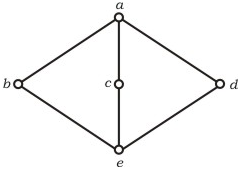# GATE | GATE-CS-2005 | Question 90

• Last Updated : 28 Jun, 2021

The following is the Hasse diagram of the poset [{a, b, c, d, e}, ≤]The poset is

(A) not a lattice
(B) a lattice but not a distributive lattice
(C) a distributive lattice but not a Boolean algebra
(D) a Boolean algebra

Explanation:

```It is a lattice but not a distributive lattice.

Table for Join Operation of above Hesse diagram

V |a b c d e
________________
a |a a a a a
b |a b a a b
c |a a c a c
d |a a a d d
e |a b c d e

Table for Meet Operation of above Hesse diagram

^ |a b c d e
_______________
a |a b c d e
b |b b e e e
c |c e c e e
d |d e e d e
e |e e e e e

Therefore for any two element p, q in the lattice (A,<=)

p <= p V q ; p^q <= p

This satisfies for all element (a,b,c,d,e).

which has 'a' as unique least upper bound and 'e' as unique
greatest lower bound.

The given lattice doesn't obey distributive law, so it is
not distributive lattice,

Note that for b,c,d we have distributive law

b^(cVd) = (b^c) V (b^d). From the diagram / tables given above
we can verify as follows,

(i) L.H.S. = b ^ (c V d) = b ^ a = b

(ii) R.H.S. = (b^c) V (b^d) = e v e = e

b != e which contradict the distributive law.
Hence it is not distributive lattice.

so, option (B) is correct. ```
My Personal Notes arrow_drop_up